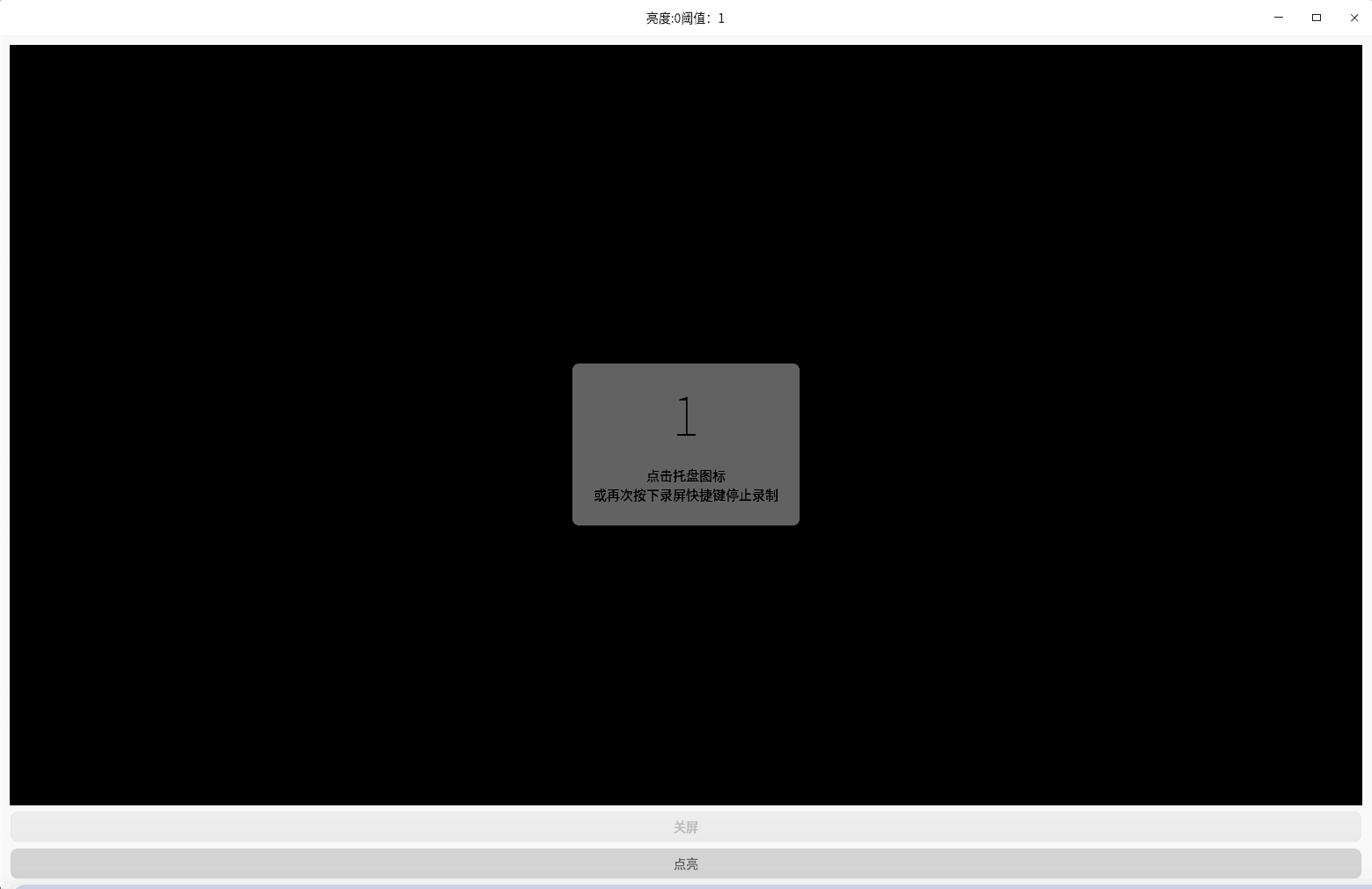## 用Qt(C++)实现如苹果般的亮屏效果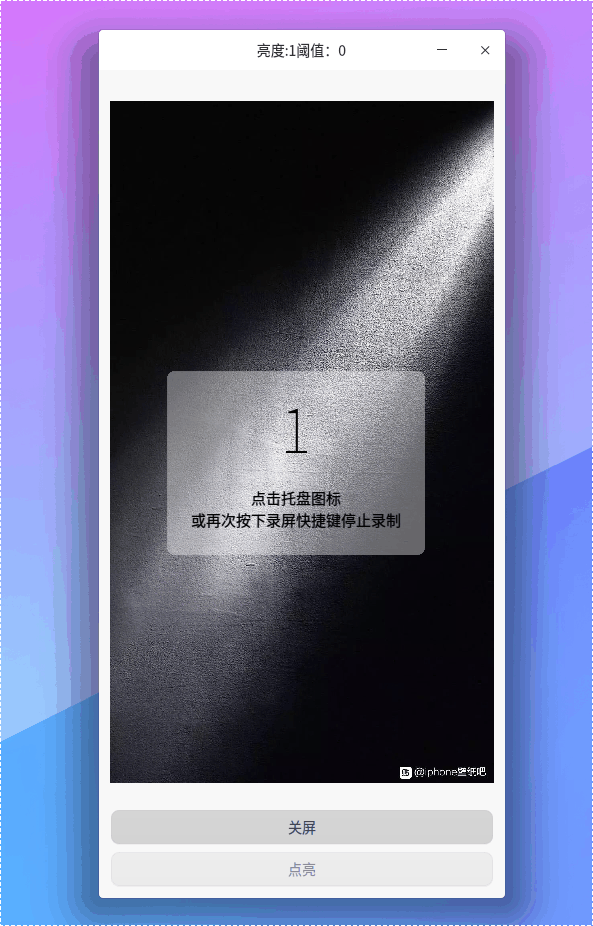## 用到

``````//该函数用于设置图片中(x,y)点的rgb值
void QImage::setPixel(int x, int y, uint index_or_rgb);
``````

## 思路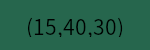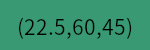## 实现

``````#include "mainwindow.h"
#include "ui_mainwindow.h"
#include <QRgb>
#include <QImage>
#include <QTimer>
#define PASS 0.3;
MainWindow::MainWindow(QWidget *parent) :
QMainWindow(parent),
ui(new Ui::MainWindow)
{
ui->setupUi(this);
double size=0.4; //图片缩放系数
p=p.scaled(p.width()*size,p.height()*size);
ui->label->setPixmap(QPixmap::fromImage(p));
speed=0;
timer=new QTimer;
timer->setInterval(1);
i=0;
connect(timer,&QTimer::timeout,[=](){
i+=speed;
MainWindow::update(i);
if(i>100 || i<0){
i=0;
timer->stop();
}
});
on_pushButton_clicked();
}

MainWindow::~MainWindow()
{
delete ui;
}

void MainWindow::update(int position) //参数为0-100，若从0开始，则逐步变亮，若从100开始，则逐步变暗
{
QImage t(p);
double bright=double(position)/100; //亮度系数
bright=(bright>1)?1:bright;
bright=(bright<0)?0:bright;

double flag=-bright+1; //渐亮需要的阈值
setWindowTitle("亮度:" + QString::number(bright)+
"阈值："+ QString::number(flag));
for(int x=0;x<p.width();x++){
for (int y=0;y<p.height();y++) {
QColor c(p.pixel(x,y));
double b=double(c.red()+c.green()+c.blue())/765; //计算当前像素的亮度
if(b>flag){ //判断亮度与阈值的大小关系
//若亮度大于阈值，将该像素乘以亮度系数
c.setRgb((int(c.red()*bright)>255)?255:int(c.red()*bright),
(int(c.green()*bright)>255)?255:int(c.green()*bright),
(int(c.blue()*bright)>255)?255:int(c.blue()*bright));
}else {
//若亮度小于阈值，则显示黑色像素，这部分会导致出现问题，后边会替换。
c.setRgb(0,0,0);
}
t.setPixel(x,y,c.rgb());//将像素写入临时变量
}
}
ui->label->setPixmap(QPixmap::fromImage(t));//将计算好的一帧显示在label上
}

void MainWindow::on_pushButton_clicked()
{
//灭
i=100;
speed=-3;
timer->start();
}

void MainWindow::on_pushButton_2_clicked()
{
//亮
i=0;
speed=3;
timer->start();
}

``````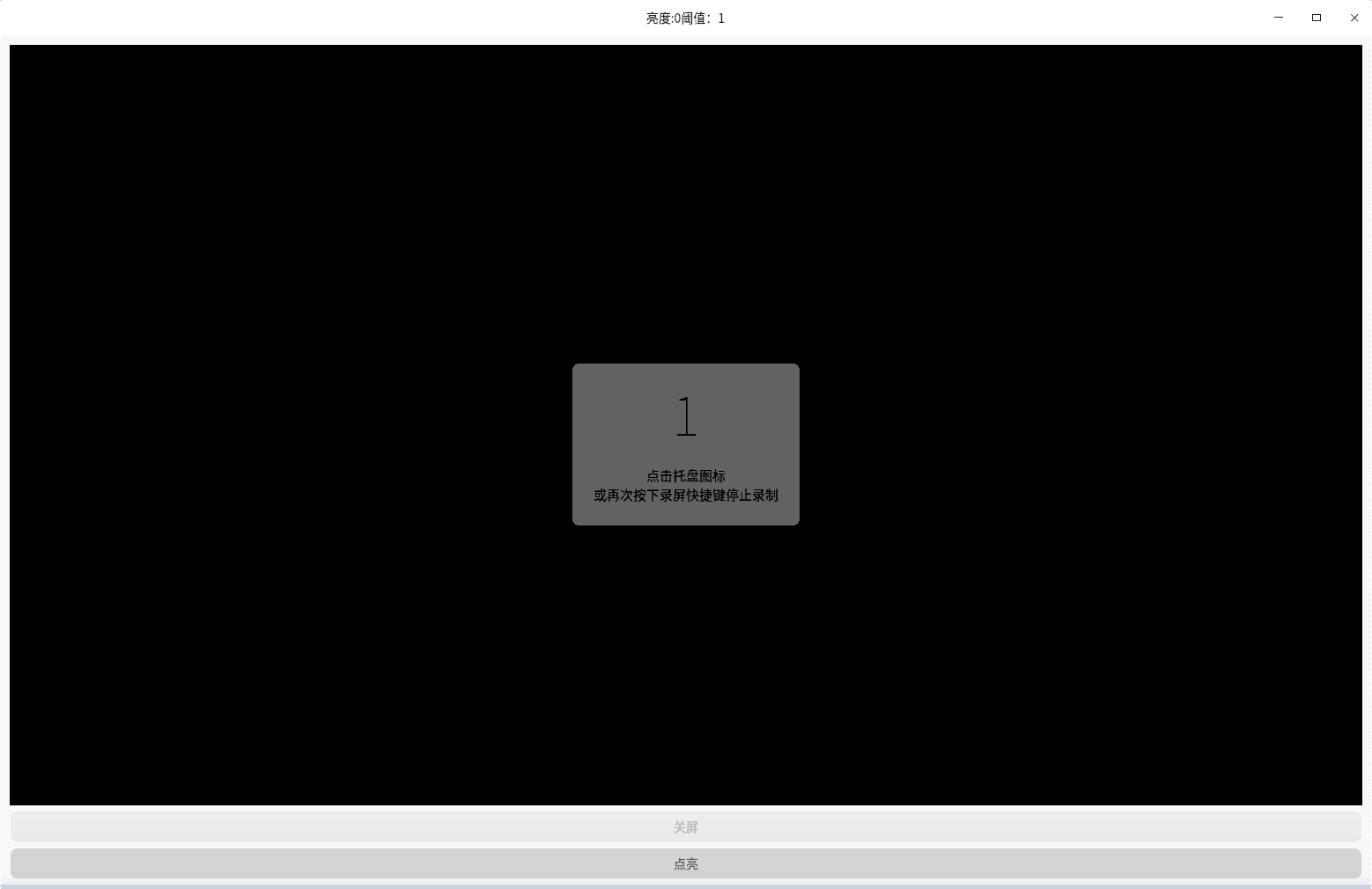## 问题

``````else {
//c.setRgb(0,0,0);
double a=1.0-(flag-b)*10; //获取加权亮度系数，亮度越大，系数越大，亮度越小，系数越小，最小到0
a=(a<0)?0:a;
c.setRgb((int(c.red()*bright*a)>255)?255:int(c.red()*bright*a),
(int(c.green()*bright*a)>255)?255:int(c.green()*bright*a),
(int(c.blue()*bright*a)>255)?255:int(c.blue()*bright*a));
}
``````